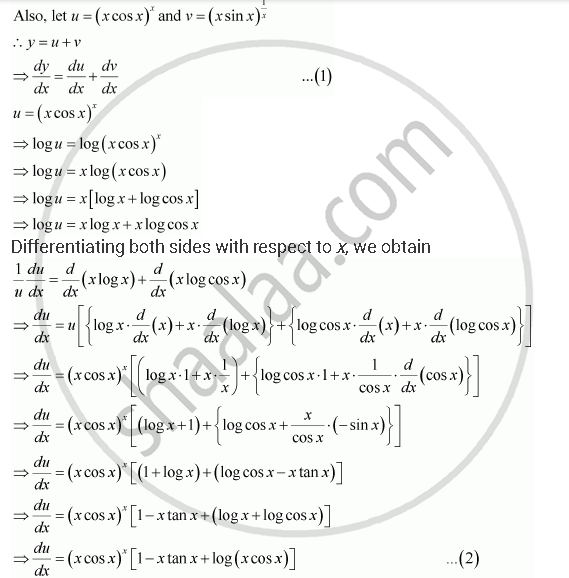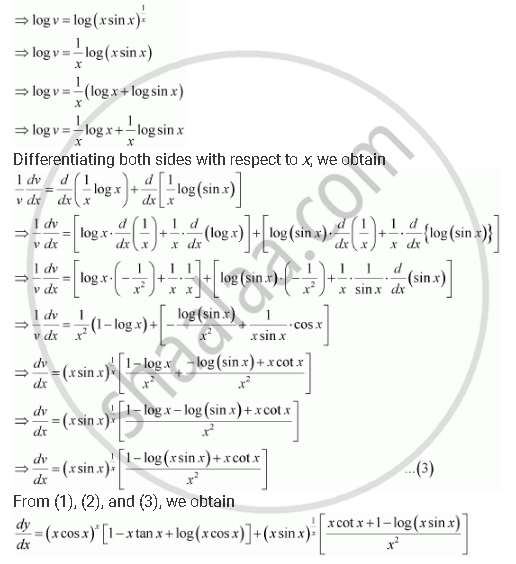Share

# Differentiate the Function with Respect To X. (X Cos X)^X + (X Sin X)^(1/X) - CBSE (Science) Class 12 - Mathematics

#### Question

Differentiate the function with respect to x.

(x cos x)^x + (x sin x)^(1/x)

#### Solution

Let y = (x cos x)^x + (x sin x)^(1/x)v= (x sin x)^(1/x)Is there an error in this question or solution?

#### APPEARS IN

NCERT Solution for Mathematics Textbook for Class 12 (2018 to Current)
Chapter 5: Continuity and Differentiability
Q: 11 | Page no. 178

#### Video TutorialsVIEW ALL 

Solution Differentiate the Function with Respect To X. (X Cos X)^X + (X Sin X)^(1/X) Concept: Logarithmic Differentiation.
S